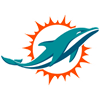2021 NFL Offensive Rookie of the Year Odds
+300
3 to 1Trevor Lawrence
18.1% implied probability

+700
7 to 1Justin Fields
9.0% implied probability

+800
8 to 1Zach Wilson
8.0% implied probability

+800
8 to 1Trey Lance
8.0% implied probability

+900
9 to 1Kyle Pitts
7.2% implied probability

+1000
10 to 1Najee Harris
6.6% implied probability

+1100
11 to 1Mac Jones
6.0% implied probability

+1200
12 to 1Ja'Marr Chase
5.6% implied probability

+1400
14 to 1DeVonta Smith
4.8% implied probability

+2000
20 to 1Jaylen Waddle
3.4% implied probability

+2200
22 to 1Travis Etienne
3.1% implied probability

+2200
22 to 1Javonte Williams
3.1% implied probability

+2500
25 to 1Trey Sermon
2.8% implied probability

+3300
33 to 1Michael Carter
2.1% implied probability

+4000
40 to 1Rashod Bateman
1.8% implied probability

+5000
50 to 1Davis Mills
1.4% implied probability

+5000
50 to 1Elijah Moore
1.4% implied probability

+5000
50 to 1Terrace Marshall
1.4% implied probability

+6000
60 to 1Rondale Moore
1.2% implied probability

+6000
60 to 1Kadarius Toney
1.2% implied probability

+8000
80 to 1Dyami Brown
0.9% implied probability

+10000
100 to 1Kyle Trask
0.7% implied probability

+10000
100 to 1Kenneth Gainwell
0.7% implied probability

+12500
125 to 1Rhamondre Stevenson
0.6% implied probability

+12500
125 to 1Pat Freiermuth
0.6% implied probability